The position (in thousands of feet) of a car driving along a straight road at time $t$ in minutes is given by the function $y = s(t)$ that is pictured below.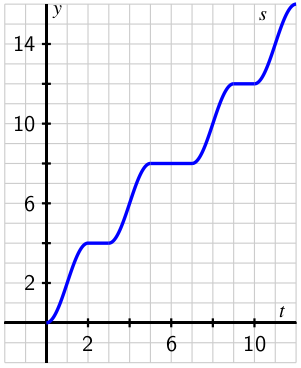Let $v(t)$ denote the velocity of the car (in thousands of feet per minute) at time $t$ (in minutes). Which graph A-F is the best representative of the derivative function $v'(t)$?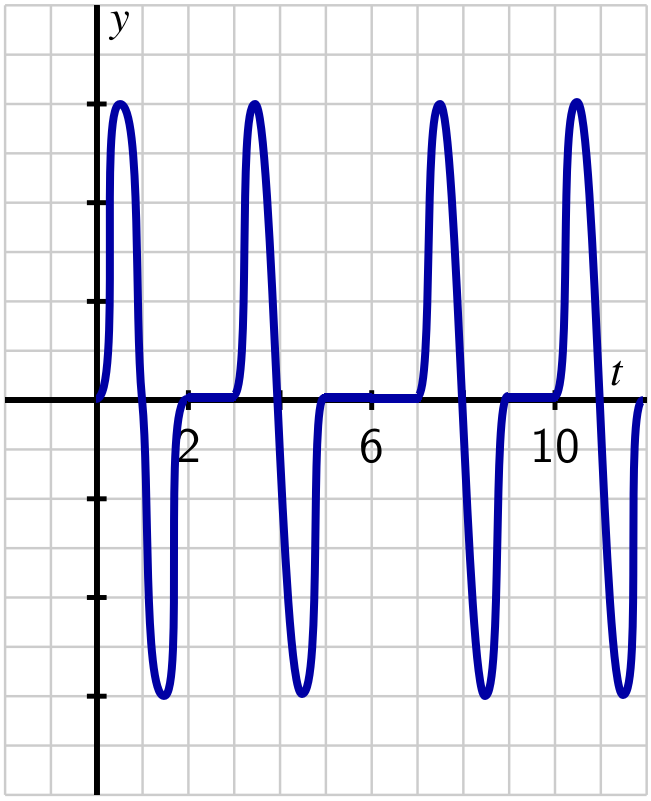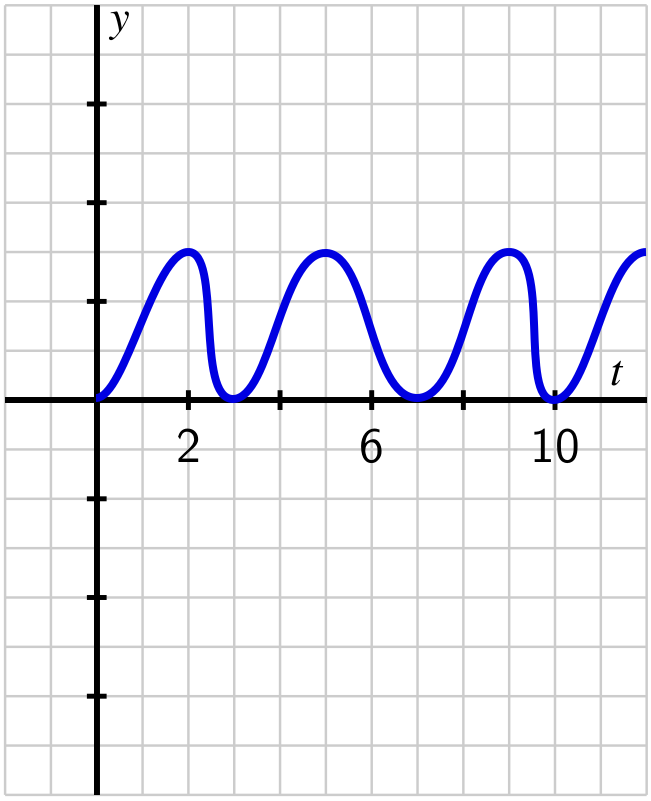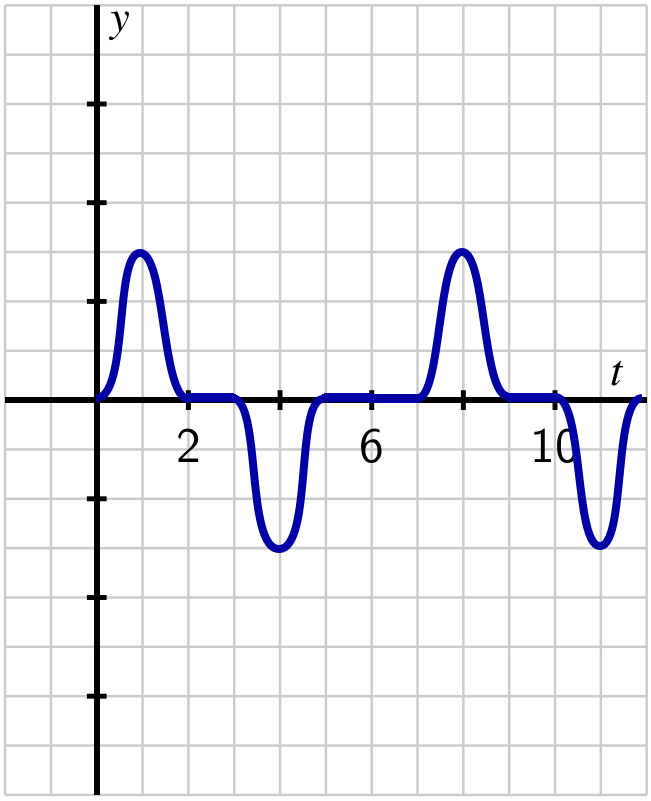A B C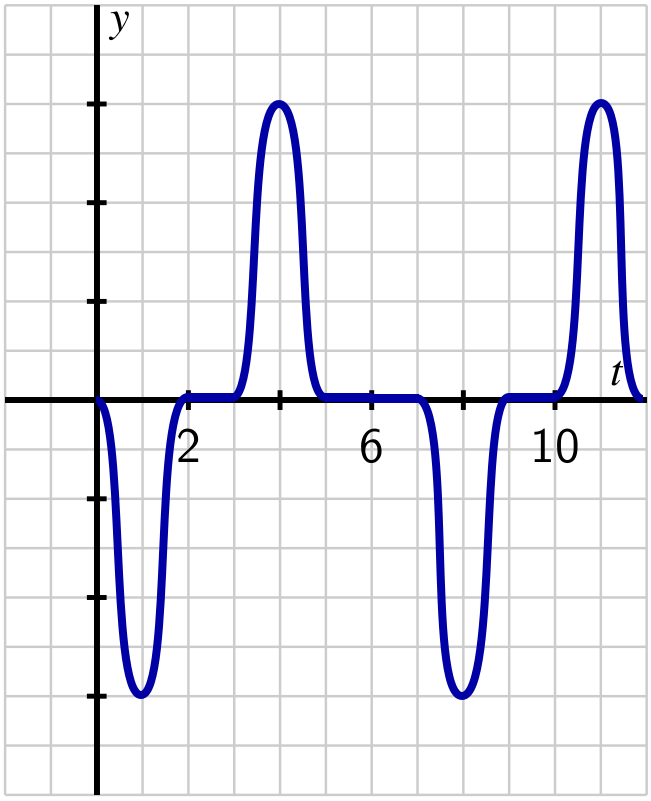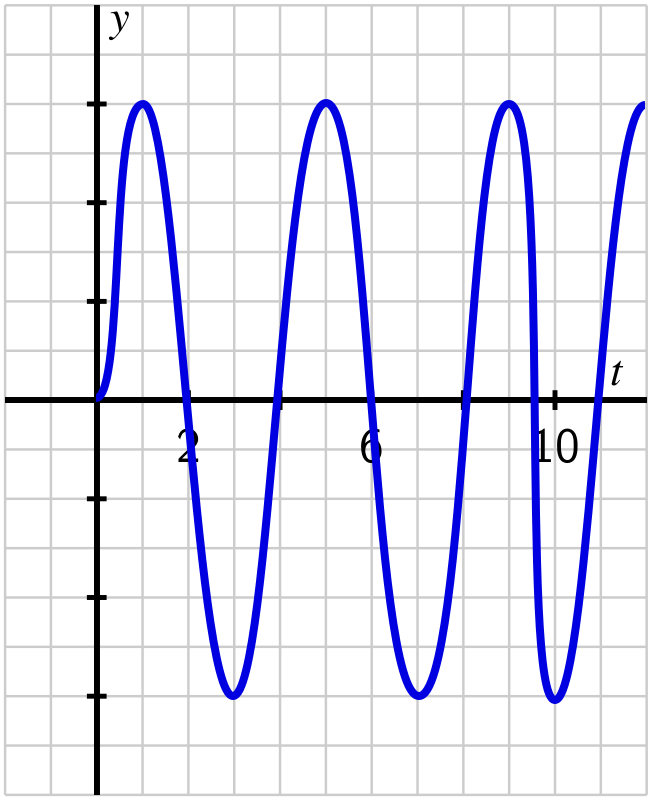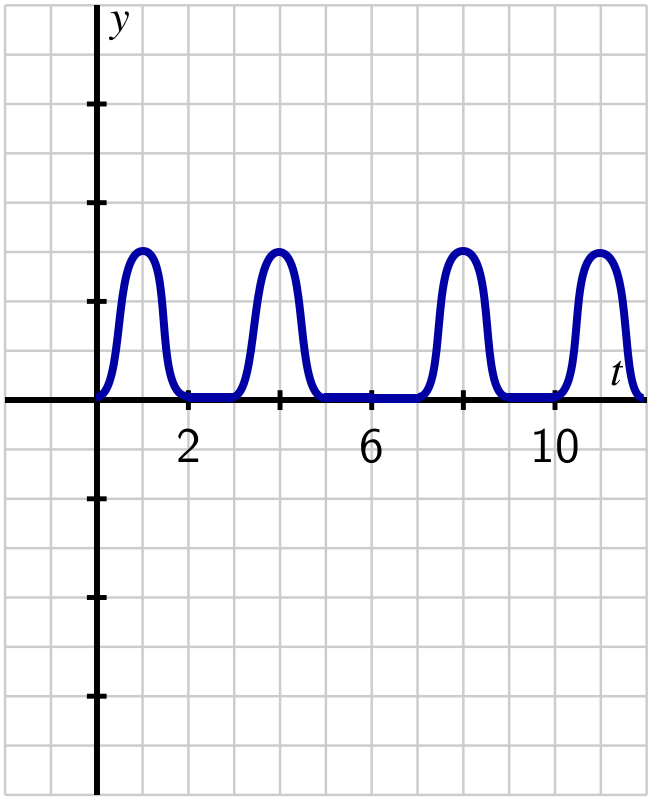D E F

(Note: the vertical axes of these graphs may have been scaled to to fit these functions. Click on a graph to enlarge it.)

Which of the following statements are true? Select all that apply.

In order to get credit for this problem all answers must be correct.